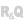HelpEditorsControls (window/page/report)ControlsTable controlTable populated programmaticallyTable control populated programmatically: WLanguageOverviewInitializing a Table control populated programmaticallyAdding rows to a Table control populated programmaticallyManaging calculated columnsModifying a row or columnOperations on rows and cellsSee alsoTable control populated programmatically: WLanguageTable control populated programmatically: WLanguage (prefix syntax)The Table control populated programmatically
 OverviewInitializing a Table control populated programmaticallyAdding rows to a Table control populated programmaticallyManaging calculated columnsModifying a row or columnOperations on rows and cellsDeleting a rowRetrieving the content of a row or cellValue of a cell in the current rowValue of a cell in a specific rowContent of the current rowContent of a specific row WINDEVWEBDEVWINDEV MobileOthersTable control populated programmatically: WLanguage Overview Here is an overview of how to handle Table controls populated programmatically in WLanguage. WLanguage includes multiple functions to handle this type of control in the code. To learn more on these functions, see the documentation. For more details, see Table control management functions. Initializing a Table control populated programmatically Rows are not created automatically in a Table control. This must be specified by calling:TableAdd or TableAddLine: adds a row at the end of the Table control. TableInsert or TableInsertLine: adds a row at the specified index or at the current index. Remark: if "Cascading input (Addition)" is not selected:The Table control contains no rows when it is created: TABLE_MyTable.Count = 0. In this case, no input is allowed and Empty is set to True.To automatically insert rows into a Table control, write the following lines of code in the control initialization event: IF TABLE_MyTable.Empty = True THEN TableAdd(TABLE_MyTable) Adding rows to a Table control populated programmatically You can add rows to a Table control:with TableAddLine and TableInsertLine: in each row, the value of each column is separated by commas.with TableAdd and TableInsert: in a row, the value of each column is separated by TAB.Managing calculated columns The calculation formula of a calculated column must be defined in the "Display a row" event of a Table control. For example: // COL_PBT: calculated column// COL_UPBT and COL_QTY: columns populated programmaticallyCOL_PBT = COL_UPBT * COL_QTY Modifying a row or column The content of the rows and columns in a Table control can be modified:by the user, by entering data directly in the columns. The changes are automatically saved in the Table control (no additional code is required). The Modified property is set to True.programmatically:with TableModify to change the content of the current row or the content of a given row.For example: TableModify(TABLE_CUSTOMER, "MOORE" + TAB + "Vince" + TAB + "Miami") Remark: you can also use the name of the Table control directly: // Modify the current rowTABLE_CUSTOMER = "MOORE" + TAB +"Vince" + TAB + "Miami"// Modify row 3TableModify(TABLE_DAY, "Wednesday" + TAB + "Off", 3) by specifying the name of the column to change the content (as for an Edit control). To modify the column of a specific row, the row number must be specified (index).For example: COL_NAME[Index] = EDT_CustomerName To modify the column of the current row, there is no need to specify the index. For example: COL_NAME = EDT_CustomerName The Modified property is set to False (it is set to True only when data is entered in the Table control). // Entry process in the COL_QTY column// COL_QTY cannot be entered, // if COL_PRODUCT is not enteredIF NoSpace(COL_PRODUCT) = "" THEN Error("The Product column must be entered first") SetFocusAndReturnToUserInput(COL_PRODUCT)END Operations on rows and cells Deleting a rowYou can delete rows by calling TableDelete. The syntax used is as follows: TableDelete([, ]) If the index is specified, TableDelete deletes the row that corresponds to that index. Otherwise, the current row is deleted. For example: TableDelete(TABLE_CUSTOMER) Deleting a row from a Table control also deletes all the values of the columns for that row.A row can be selected with TableSelectPlus.The syntax used is as follows: TableSelectPlus([, ]) Retrieving the content of a row or cellThe content of a Table control populated programmatically can be retrieved:for the entire row. cell by cell. Value of a cell in the current rowTo retrieve the value of a column (or cell) for the current row, the syntax is the same as for a simple edit control. = Example: // COL_QTY is a column of the Table controlIF COL_QTY < 10 THEN Info("Insufficient quantity")END Value of a cell in a specific rowTo retrieve the value of a column that is not in the current row, you must specify the index of the row. = [] For example: // Add the total price before tax (PBT) for all the order linesTotalPrice = 0FOR Index = 1 _TO_ TABLE_ORDER.Count TotalPrice = TotalPrice + COL_PBT[Index]END Content of the current rowTo retrieve the content of the current row, use the following syntax: = Content of a specific rowTo get the content of the row at index , use the following syntax: = [] Remark: The index of the current row is returned by TableSelect. For example: // Retrieve row #10 in the TABLE_CUSTOMER controlCurrentRow = TABLE_CUSTOMER // Name of selected customerCustomerName = COL_NAME[TableSelect(TABLE_CUSTOMER)] // Retrieve the current row in the TABLE_CUSTOMER controlCurrentRow = TABLE_CUSTOMER Minimum version required Version 9 This page is also available for…